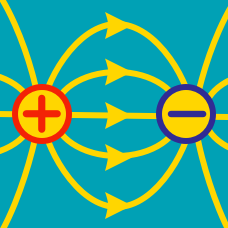Electricity and Magnetism

# Electrostatics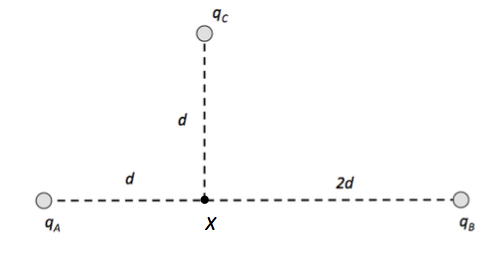As shown in the above figure, the point charges $q_A = 4 \text{ C}$ and $q_B$ produce potential $V$ at the point $X,$ which is away from $q_A$ by a distance of $d$ and away from $q_B$ by a distance of $2d.$ If the point charge $q_C$ is away from $X$ by a distance of $d$ and the total potential at $X$ is $-3V,$ what is the sum of the point charges $q_A,$ $q_B$ and $q_C?$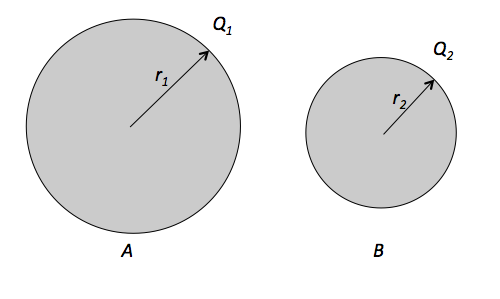There are two charged metal spheres, as shown in the above figure. The sphere $A$ with radius $r_1 =16 \text{ cm}$ is charged with charge $Q_1 =32 \text{ C} ,$ and the sphere $B$ with radius $r_2 =8 \text{ cm}$ is charged with charge $Q_2 = 4 \text{ C}.$ If the two spheres touch each other, what is the final charge of the sphere $A?$

Two identical, equally charged metal spheres $A$ and $B$ with radius $3 \text{ m}$ repel each other with a force of $F_{AB} = 5 \times 10^{-5} \text{ N}.$ A third identical, uncharged sphere $C$ touches $A,$ and then is placed at the midpoint of $A$ and $B.$ Determine the magnitude of net electrostatic force on $C?$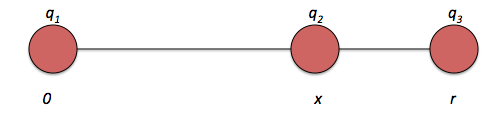Three positively charged particles lie on a straight line. Their charges are $q_1= 4 \text{ C}, q_2 =5 \text{ C}$ and $q_3=16 \text{ C},$ with positions $x_1 = 0,$ $x_2$ and $x_3 = r = 24 \text{ m}$. The equilibrium point is placed between $q_1$ and $q_3.$

When $q_1$ and $q_3$ are fixed, and $q_2$ is movable, find the equilibrium point for $q_2.$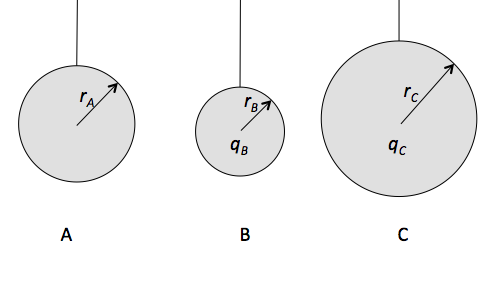As shown in the above diagram, there are three electrically neutral metal spheres: metal sphere $A$ with radius $r_A=4 \text{ cm},$ metal sphere $B$ with radius $r_B = 2 \text{ cm}$ which is positively charged with charge $q_B = 6.0 \text{ C} ,$ and metal sphere $C$ with radius $r_C = 6 \text{ cm}$ which is negatively charged with charge $q_C = -10.0 \text{ C}.$ If $B$ touches $A$ and then touches $C,$ what is the final charge of sphere $B?$

×